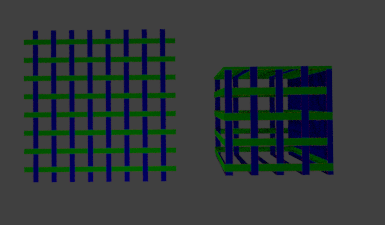# Correct Transparency in OSL

I am reviewing my OSL notes and still unsure about one thing: Transparency.

My reference is this note by Steve May on RSL:

So far in my OSL Shader study, I only care about the procedural texture part. But I want to further understand and properly write code especially when “Layering, Masking, and dealing with Opacity”.

How do we actually implement the Os (related to Opacity) in OSL?

Below is my code so far.

``````#include "/Users/jimmygunawan/Desktop/rmannotes.sl"

(
vector Pos = P,
color ColA = color (0.3, 0.3, 0.3), // grey
color ColB = color(0,0,1), //blue
color ColC = color(0,1,0), // green
output color ColOut = 0,
output color TransOut = 0
)
{

float s = Pos;
float t = Pos;

color surface_color, layer_color;
color surface_opac, layer_opac;

float ss, tt;
point Nf, V;

/* background layer (layer 0) */
surface_color = ColA;
//surface_opac = Os;

/* layer 1 */

ss = repeat(s,4);
tt = repeat(t,4);

layer_color = ColB;
layer_opac = pulse(0.35, 0.65, 0.2, ss);  /* vertical stripe */
layer_opac *= 0.75;
surface_color = blend(surface_color, layer_color, layer_opac);
surface_opac = union(surface_opac, layer_opac);

/* layer #2 */
ss = repeat(s,4);
tt = repeat(t,4);

layer_color = ColC;
layer_opac = pulse(0.35, 0.65, 0.02, tt);
layer_opac *= 0.5;
surface_color = blend(surface_color, layer_color, layer_opac);
surface_opac = union(surface_opac, layer_opac);

// OUTPUT
ColOut = surface_color;
TransOut = surface_opac; // this is wrong

}

``````

Further on… I don’t know if this is correct or not. Maybe there is a better way to think of this.

#include “/Users/jimmygunawan/Desktop/rmannotes.sl”

(
vector Pos = P,
color ColA = color(1,0,0),
color ColB = color(0,1,0),
color ColC = color(0,0,1),
float Fuzz = 0.05,
float Freq = 1.0,
output color ColOut = 0,
output color Fac = 0
)
{
float s,t;
s = Pos;
t = Pos;

``````float ss,tt;
ss = repeat(s,Freq);
tt = repeat(t,Freq);

float col, row;
col = whichtile(s, Freq);
row = whichtile(t, Freq);

// get base color
color surface_color;
surface_color = ColA;

//
color transparency_color;
transparency_color = color(1,1,1); // BLACK

// This is for COLOR OUT
if (even(row) && odd(col) || even(col) && odd(row)) {

// vertical bar layer
float f;
f = pulse(0.35,0.65, Fuzz, ss);
surface_color = blend(surface_color, ColB, f);

// horizontal bar layer
f = pulse(0.35,0.65, Fuzz, tt);
surface_color = blend(surface_color, ColC, f);

} else {

// vertical bar layer
float f;
f = pulse(0.35,0.65, Fuzz, tt);
surface_color = blend(surface_color, ColC, f);

// horizontal bar layer
f = pulse(0.35,0.65, Fuzz, ss);
surface_color = blend(surface_color, ColB, f);

}

// This is for TRANSPARENCY OUT
if (even(row) && odd(col) || even(col) && odd(row)) {

// vertical bar layer
float f;
f = pulse(0.35,0.65, Fuzz, ss);
transparency_color = blend(transparency_color, color(0,0,0), f);

// horizontal bar layer
f = pulse(0.35,0.65, Fuzz, tt);
transparency_color = blend(transparency_color, color(0,0,0), f);

} else {

// vertical bar layer
float f;
f = pulse(0.35,0.65, Fuzz, tt);
transparency_color = blend(transparency_color, color(0,0,0), f);

// horizontal bar layer
f = pulse(0.35,0.65, Fuzz, ss);
transparency_color = blend(transparency_color, color(0,0,0), f);

}

ColOut = surface_color;
Fac = transparency_color;
``````

}

This is the BLEND file.

http://www.pasteall.org/blend/26764

You need to “include” the:
rmannotes.sl

in order to make the OSL working.